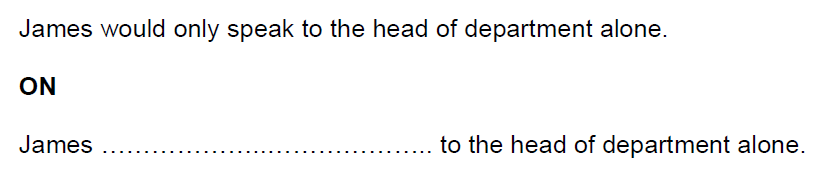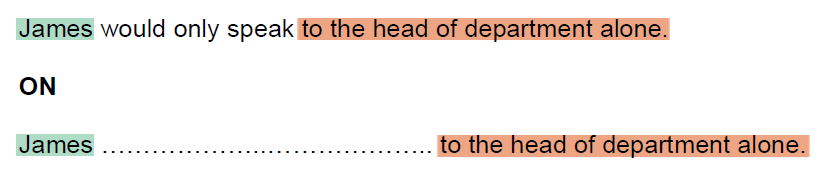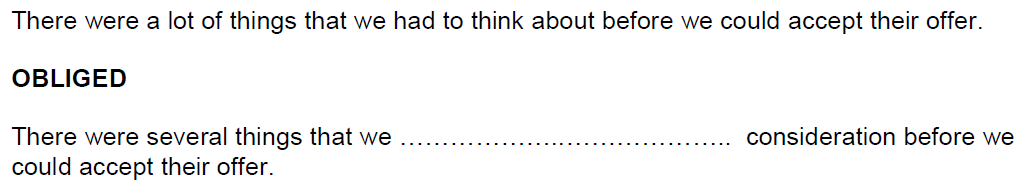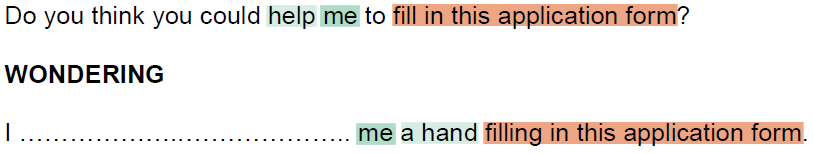Now that you have a strategy for time management during the Use of English section of the exam, let’s look at exactly how to approach part 5.  Remember, this is the only section of the exam where you can get one point even if your answer is not 100% correct (in that case you will get two points).  This means that you can try and get at least one point even if you don’t think you can get both of them.  Typically, each correct answer has a grammar component and a vocabulary component.  If you think of it this way, you might be able to find some extra points more easily.

The instructions (which you should memorise… how many words are you allowed to write?) tell you that the sentences should have “a similar meaning”.  Really, in my experience, similar is not going to be good enough.  The correct answer will be almost exactly the same meaning as the original, so you will need to be very careful.  In order to see what you need to change, it will help to see all of the things that have stayed the same.  You can underline or circle text when you take the exam, I’ve just used colours to highlight the text so it will be easy to see.

Here is the example from the official CAE practice test:You can see that almost everything is the same, but let’s highlight them anyway.  For me, this just helps me be more confident that I’ve seen everything.So let’s imagine that we just don’t have any idea how to answer this particular question.  We can still use what we know about English to get at least one point.  After we highlight the parts of the sentences that are the same, we can clearly see that we need to find the words to express “would only speak”, and that we need to use the word “on”.  We can see that in the second sentence, there is no verb, so we can assume that ”speak” will somehow be part of our answer.  If we think about “on”, it would be strange to make a phrase like “speak on… to the head of the department”, so we can probably assume that “on” will come before some form of “speak”.  What form of the verb follows prepositions?  Always the –ing form: here is the grammar component of the answer.  So if we just write “on speaking” on the answer sheet, we would get one point!  If we understand the use of “would” in the first sentence (as a way of stating demands or refusals, for example, “I wouldn’t eat broccoli when I was a child.”), we could also find the correct vocabulary item to make the full correct answer: “insisted on speaking”.

Click on the tabs below to see two more questions from the practice exam and the way that I would try to solve them if I didn’t know the answer. If you can’t see the practice questions clearly, you can click the images for a larger size.

Here is one of the questions from the official CAE practice test. Try it yourself first, identify what is the same so that you can focus on what you need to change. Then try and identify what is the grammar part of the answer and what is the vocabulary part, and finally, try and find the full correct answer.Now let’s do it together. I’ve highlighted everything that is the same (or almost exactly the same).OK, last one—can you find the grammar point and the vocabulary point? Isolate the part of the sentence you need to change and then try and find the solution. When you’re done, check my suggested method and the correct answer.What do we notice when we compare the two sentences? The biggest difference, in my opinion, is that the first sentence is a question while the second is a statement. This means there could be a major grammatical difference. Other than that, the two sentences are not as similar as the others we’ve looked at during this exercise. Let’s take a careful look:The ends of the sentences are the same, “me” appears in both sentences, and I gave a lighter highlight to the words “help” and “a hand” because they seem to be related even though they are not exactly the same. Do you think this might be the vocabulary point? What’s the expression with “a hand” that means the same as “help me”?

For the grammar point, we need to look at the phrase that we are trying to replace: “Do you think you could…” When do we use this phrase and why? What other phrases do we use in a similar context? And what tense and verb forms do we use? Finally, try and guess where in the sentence the points will be divided (where will this symbol ][ appear in the answer?). Make sure you have written enough so that you get at least one point, if you know it!

The correct answer is: was wondering if/whether ][ you could/would/might lend/give (The words separated by a slash (/) are all acceptable in the answer, as long as you have only written one of them.)

I hope this little practice exercise has helped you understand this part of the exam better and will give you more confidence when taking the test.  Don’t forget to take plenty of practice tests, and good luck!